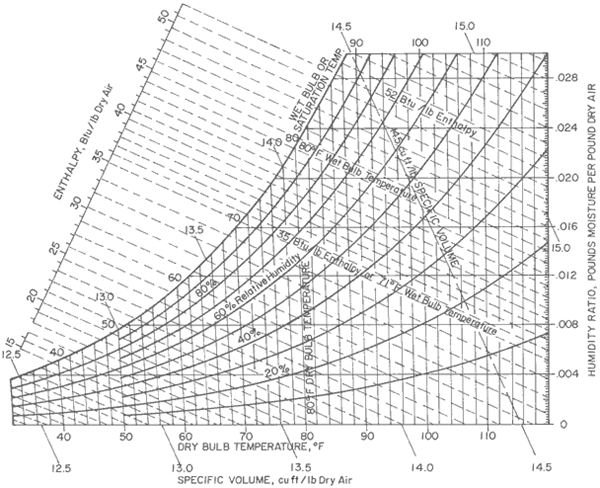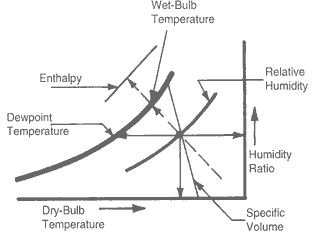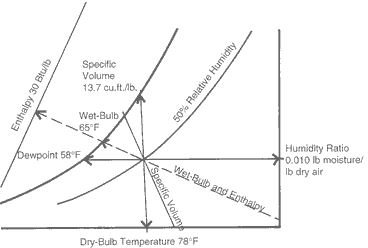# How to Use a Psychrometric Chart: Lines and Curves

Page content

## Various Lines and Curves in the Psychrometric Chart

All the properties of air indicated in the psychrometric chart are calculated at standard atmospheric pressure. For other pressures, relevant corrections have to be applied. The psychrometric chart looks like a shoe. The various lines shown in the chart are as follows (please refer the fig). In the first part of this series we saw representation of DB, WB, DP temperature and moisture content on the pschrometric chart. Now we’ll explore some more parameters.1. Relative Humidity (RH) Lines:

The RH lines are the curves extending from the lower left to the upper right part of the psychrometric chart. The different curves of RH indicate different values of humidity measured in percentage. The value of RH reduces from left towards right. The extreme left curve along the shoe indicates RH of 100% and is also called the saturation curve because the condition of air along this line is fully saturated no matter what the DB and WB temperatures are. Along the saturation curve all the three temperatures, the DB, WB and DP are same.

1. Total Enthalpy of the Air:

The total enthalpy of the air comprises of the sensible heat and the latent heat. The enthalpy scale in the psychrometric chart is located outside the main body. The constant enthalpy lines extend from the enthalpy scale down toward the right side of the chart. To find the value of the enthalpy of the any given air, one should know its WB temperature. The constant enthalpy line passing through the given value of the WB temperature indicates the enthalpy of the air.

1. Specific Volume of the Air:

The specific volume of air is an important parameter since one has to consider the amount of air to be handled by the cooling coil, cooling fan, etc and accordingly the rating of cooling coil and fan has to be found out. From the psychrometric chart we can find out the specific volume of the required air and find the total volume of the required air by multiplying it with the total weight of the air required. The constant specific lines start from the saturation curve and drop down with the slight angle to the vertical lines. They indicate the value in either m3/kg or ft3/lb.

If any of the two values from DB, WB, DP temperature and the relative humidity are known, all other values can be easily found from the psychrometric chart, without having to carry out any calculations.

## Example Showing How to Use Psychrometric Chart

Consider an example of air where the given values of DB and WB temperature are 78 degrees F and 65 degrees F respectively. We will find out various values from these given values of DB and WB temperatures.

1. On the psychrometric chart locate value 78oF on the DB temperature scale located at the bottom of the chart.

2. Locate WB temperature of 65oF on the saturation curve scale.

3. Extend the vertical line (constant DB temperature line) from 78o and the diagonal line (constant WB temperature line) from 65oF and get the point of intersection of the two lines, which indicates the condition of the given air.

4. Find out the values of various parameters at this point. The relative humidity line passing though this point indicates RH of 50%. The horizontal line passing though this point and meeting the saturation curve indicates DP temperature of 58oF. The specific volume of this air is 13.7 ft3/lb and the enthalpy of air is 30 Btu/lb.1. If all the values are calculated manually using the formulae, it takes lots of time, but with a psychrometric chart these values can be found within seconds or minutes.

2. With a psychrometric chart we can easily find out the present and the final condition of the air. Say if the air is cooled from 100oF to 75oF, all we have to do is draw the horizontal line to locate the initial and final condition of the air.

3. A psychrometric chart is very useful in carrying out heat load or cooling load calculations.

## Reference

1. Book: Principles of Refrigeration by Roy J. Dossat

2. Book: Basic Refrigeration and Air Conditioning by P N Ananthanarayan

## This post is part of the series: Psychrometric Chart

This is the series of articles that describes psychrometric chart and various psychrometric processes like sensible heating, sensible cooling, humidification, de-dumidification, evaporative cooling etc.